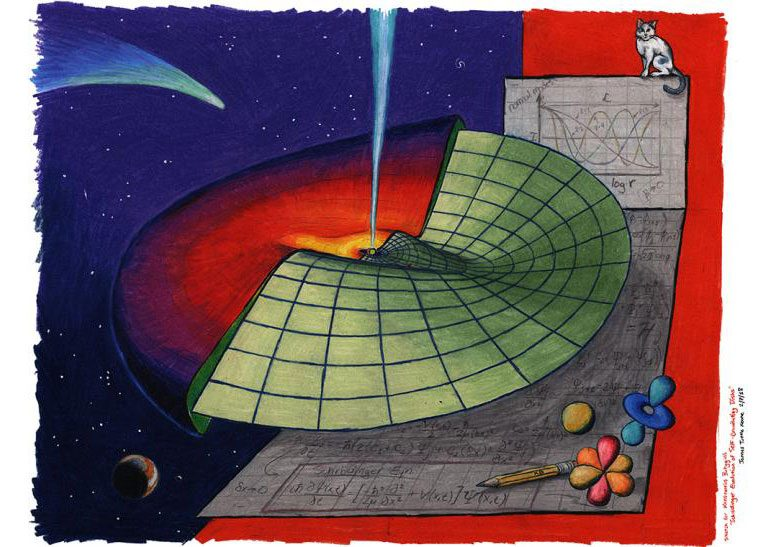# Schrödinger Equation Describes the Evolution of Astronomical StructuresSchrödinger in Space: An artist’s impression of research presented in Batygin (2018), MNRAS 475, 4. Propagation of waves through an astrophysical disk can be understood using Schrödinger’s equation – a cornerstone of quantum mechanics. Credit: James Tuttle Keane, California Institute of Technology

Quantum mechanics is the branch of physics governing the sometimes-strange behavior of the tiny particles that make up our universe. Equations describing the quantum world are generally confined to the subatomic realm—the mathematics relevant at very small scales is not relevant at larger scales, and vice versa. However, a surprising new discovery from a Caltech researcher suggests that the Schrödinger Equation—the fundamental equation of quantum mechanics—is remarkably useful in describing the long-term evolution of certain astronomical structures.

The work, done by Konstantin Batygin (MS ’10, PhD ’12), a Caltech assistant professor of planetary science and Van Nuys Page Scholar, is described in a paper appearing in the March 5 issue of Monthly Notices of the Royal Astronomical Society.

Massive astronomical objects are frequently encircled by groups of smaller objects that revolve around them, like the planets around the sun. For example, supermassive black holes are orbited by swarms of stars, which are themselves orbited by enormous amounts of rock, ice, and other space debris. Due to gravitational forces, these huge volumes of material form into flat, round disks. These disks, made up of countless individual particles orbiting en masse, can range from the size of the solar system to many light-years across.

Astrophysical disks of material generally do not retain simple circular shapes throughout their lifetimes. Instead, over millions of years, these disks slowly evolve to exhibit large-scale distortions, bending and warping like ripples on a pond. Exactly how these warps emerge and propagate has long puzzled astronomers, and even computer simulations have not offered a definitive answer, as the process is both complex and prohibitively expensive to model directly.

While teaching a Caltech course on planetary physics, Batygin (the theorist behind the proposed existence of Planet Nine) turned to an approximation scheme called perturbation theory to formulate a simple mathematical representation of disk evolution. This approximation, often used by astronomers, is based upon equations developed by the 18th-century mathematicians Joseph-Louis Lagrange and Pierre-Simon Laplace. Within the framework of these equations, the individual particles and pebbles on each particular orbital trajectory are mathematically smeared together. In this way, a disk can be modeled as a series of concentric wires that slowly exchange orbital angular momentum among one another.

As an analogy, in our own solar system one can imagine breaking each planet into pieces and spreading those pieces around the orbit the planet takes around the sun, such that the sun is encircled by a collection of massive rings that interact gravitationally. The vibrations of these rings mirror the actual planetary orbital evolution that unfolds over millions of years, making the approximation quite accurate.

Using this approximation to model disk evolution, however, had unexpected results.

“When we do this with all the material in a disk, we can get more and more meticulous, representing the disk as an ever-larger number of ever-thinner wires,” Batygin says. “Eventually, you can approximate the number of wires in the disk to be infinite, which allows you to mathematically blur them together into a continuum. When I did this, astonishingly, the Schrödinger Equation emerged in my calculations.”

The Schrödinger Equation is the foundation of quantum mechanics: It describes the non-intuitive behavior of systems at atomic and subatomic scales. One of these non-intuitive behaviors is that subatomic particles actually behave more like waves than like discrete particles—a phenomenon called wave-particle duality. Batygin’s work suggests that large-scale warps in astrophysical disks behave similarly to particles, and the propagation of warps within the disk material can be described by the same mathematics used to describe the behavior of a single quantum particle if it were bouncing back and forth between the inner and outer edges of the disk.

The Schrödinger Equation is well studied, and finding that such a quintessential equation is able to describe the long-term evolution of astrophysical disks should be useful for scientists who model such large-scale phenomena. Additionally, adds Batygin, it is intriguing that two seemingly unrelated branches of physics—those that represent the largest and the smallest of scales in nature—can be governed by similar mathematics.

“This discovery is surprising because the Schrödinger Equation is an unlikely formula to arise when looking at distances on the order of light-years,” says Batygin. “The equations that are relevant to subatomic physics are generally not relevant to massive, astronomical phenomena. Thus, I was fascinated to find a situation in which an equation that is typically used only for very small systems also works in describing very large systems.”

“Fundamentally, the Schrödinger Equation governs the evolution of wave-like disturbances.” says Batygin. “In a sense, the waves that represent the warps and lopsidedness of astrophysical disks are not too different from the waves on a vibrating string, which are themselves not too different from the motion of a quantum particle in a box. In retrospect, it seems like an obvious connection, but it’s exciting to begin to uncover the mathematical backbone behind this reciprocity.”

Publication: Konstantin Batygin, “Schrödinger evolution of self-gravitating discs,” MNRAS, 2018; doi:10.1093/mnras/sty162

#### 1 Commenton "Schrödinger Equation Describes the Evolution of Astronomical Structures"

1.Joe Milosch | January 3, 2021 at 2:42 am | Reply

Its more than coincidental that Schrodinger’s Equation should mimic what we see in space-time. There is a beautiful wave-like nature to all existence. See Time, not Space.
I’ve read that there is a matrix form of the wave equation, I wonder how well these solutions satisfy the equivalent Matrix equation?